Next: Oscillatory LFP Up: The Role of Lateral Previous: Introduction

# Dynamic Pattern Formation, Spontaneous Activity and Variability of Spike Trains

The model consists of a cyclic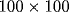lattice of units characterized by a voltage variable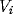. Each unit models a nerve cell as a simple RC circuit (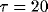msec) with the addition of a reset mechanism. Once a unit reaches a threshold voltage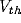, it emits a pulse that is transmitted in one iteration (1 msec) to connected neighboring units, and the potential is reset by subtracting the voltage threshold. The unit dynamics are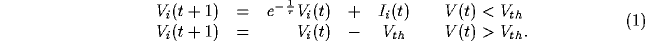where the current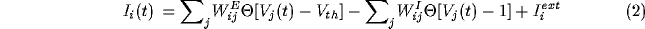represents the coupling of the unit to nearby unit through excitatory connections (matrix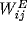) and inhibitory connections (matrix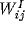). Additional input is provided by an independent external current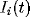. Such a model, composed of the ``integrate-and-fire''  units with the dynamics described in eq. 1, is functionally similar to a recently studied model of self-organized criticality for earthquakes .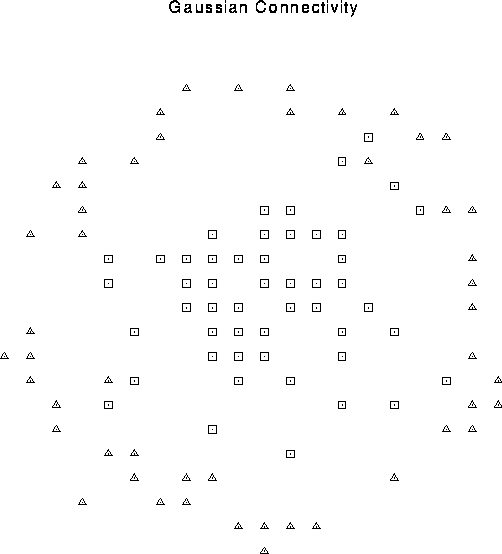Figure 1: Center surround connectivity pattern. The cell (not shown) at the center of the rectangular array is connected in a probabilistic manner to units within a given distance determined by a Gaussian distribution with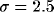lattice constants. These short-range connections are excitatory (squares). The center cell also inhibits a fixed fraction of cells on an annulus 8 and 9 lattice constants away (triangles). During a particular simulation, the connectivity pattern is fixed, although the exact synaptic weight varies stochastically.

Based on physiological evidence, the pattern of local excitatory and inhibitory connections is center-surround, for the validity of this assumption can be found. Hess and coworkers  found that iontophoretic application of the excitatory agonist glutamate to visual cortex of anesthetized cats induces excitation of neurons within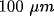of the application site and distant inhibition at distances between 100 and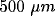. Similar studies in rat somatosensory cortical slice preparations  confirm the general pattern of an inhibitory surround enclosing the excited region. Each unit is excitatorily connected to N = 50 units chosen from a Gaussian probability distribution oflattice constants, centered at the unit's position (see Fig. 1). Likewise, N inhibitory connections per unit are chosen from a uniform probability distribution on a ring eight to nine lattice constants away. The weight of the excitation and inhibition, in units of voltage threshold, is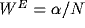and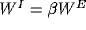. External input is modeled independently for each cell as a Poisson process of excitatory pulses of magnitude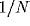, arriving at a mean rate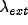.

Scaling the relative degree of inhibition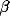while keeping the sum of excitation and inhibition constant leads to a transition from a spatially homogeneous state to a clustered activity state. This transition can be understood using a mean-field description of the dynamics, where we write the pulse rate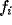as a function of the averaged currents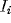: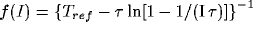, where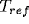is the minimum dead time between pulses. In this approximation, the dynamics associated with eq. 1 simplify to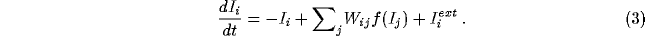The homogeneous solution is stable only if the connectivity pattern satisfies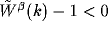for all k, where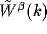is the Fourier transform of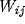. As one increases the relative strength of inhibition, clusters of high firing activity develop. The clusters form a hexagonal grid across the network, as illustrated in Fig. 2a. Clusters do not remain stationary but pursue a stochastic motion over the layer (Fig. 2b).

Figure 2: (click on the image to view a larger version) On the left, the summed activity for the network over 50 msec of simulation is shown for model parameters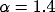,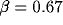, and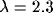inputs per msec. Lighter shades denote higher discharge rates (maximum rate 120 Hz). Note the nearly hexagonal pattern of clusters or ``hot spots" of activity. On the right, we illustrate the motion of a typical cluster. Each vertex in the graph represents a tracked cluster's position averaged over 50 msec. The diffusion constant is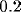lattice units/msec.

The level of external input also influences the spatial pattern; for even higher values of input the clusters merge into stripes. The transition from a homogeneous state to hexagonal clusters to stripes is generic to many nonequilibrium systems in fluid mechanics, nonlinear optics, reaction-diffusion systems, and biology [8,9,12,36]. In the mean-field description, the dynamics (3) are subject to a Lyapunov potential function  so that the system must relax to a stable state---persistent dynamics are ruled out.

However, the mean-field equations [1,52,53] do not capture the essential dynamics of pulse-coupled networks responsible for the spatial pattern's metastability. Partial synchronization inside local populations induce large fluctuations which destabilize the mean-field pattern. Indeed, simulations performed with constant (instead of stochastic) input and with isotropic connectivity reveal that the system is intrinsically chaotic. These fluctuations allow clusters of high activity to diffuse throughout the system, as can be seen from the cluster's trajectory in Fig. 2b. Repulsive interactions with surrounding clusters generally constrain the motion of a cluster to remain within a certain radius. This vibratory motion is occasionally punctuated by longer-range diffusion. If one tracks the activity of individual cells during a period of time, one observes large fluctuations leading to high variability in interspike intervals and in the total number of spikes, which are due to the fact that even under stationary stimulation the system generates its own rate fluctuations.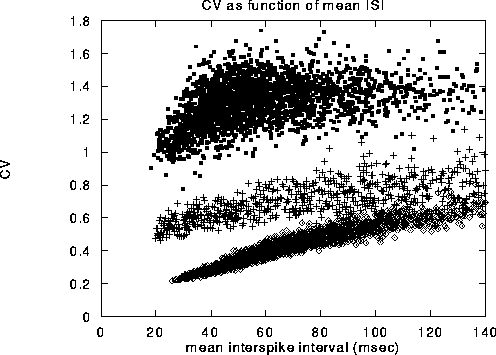Figure 3: Coefficient of variation,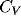, of a representative range of cells shown against the average time between spikes, that is, the inverse of the mean firing rate. The solid dots in the upper cloud are from our model. The crosses in the middle cloud represent the behavior when all network effects are eliminated (i.e.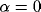), and units receive both an excitatory and an inhibitory stream of external Poisson input, with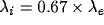. Without inhibition, the associatedvalues are reduced by a factor of about 2. The diamonds in the lowest cloud are from cells in a random network with sparse, non-local connections that have no organized topography. The same number of inhibitory and excitatory connections, 50, were used as in the local center-surround connection scheme.

The coefficient of variation () computed for all the cells in the network, over a 400 sec long simulation are displayed in Fig. 3 Note that almost all values ofare on the order of one or larger. The pattern of observed's reproduces qualitatively thevalues measured for cells in cortical areas V1 and MT in the awake monkey responding to bars and to clouds of moving dots .

The coefficient of variation does not contain enough information, however, to fully characterize the statistical properties of the spike train. In order to obtain a more complete characterization of those properties, we computed the inter-spike-interval (ISI) distribution and the cross-correlation function of spike trains (Fig. 4). We find that the ISI distribution decays as a power law in t with exponent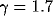over one decade (from 25 to 300 msec). The correlation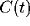decays slowly via a power law with exponent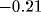, as shown in Fig. 5. At the upper temporal cutoff of 300 msec, the correlation function reaches the baseline of chance coincidence. The power spectrum at low frequencies has a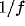form consistent with the correlation and decays as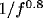(see, for more details ).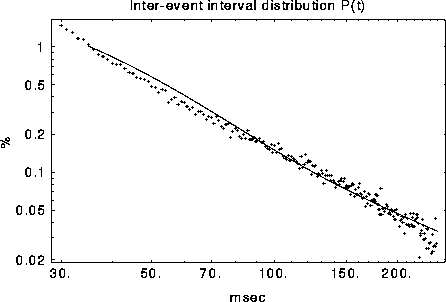Figure 4: The average ISI distribution for 19 cells. Superposition of exponential distributions from the cluster frame of reference (solid line) results in a power law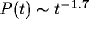in the fixed unit frame.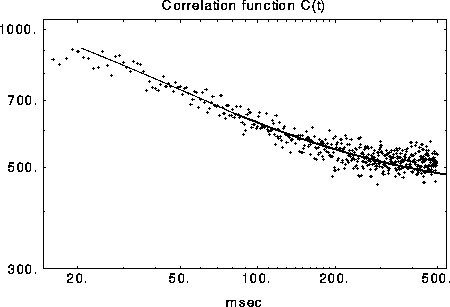Figure 5: Measured and predicted (solid line) correlationon a log-log scale. The correlation function decays as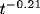due to the slow diffusion of activity clusters.

Such power-law distributions of the ISI and correlation functions have been reported in neurons from the somatosensory cortex , in the auditory pathway [49,50], in the mesencephalic reticular formation  and in visual cortex . One should note that such power-law functions are not explained by the variability of spike-trains described by Poisson processes (which have exponentially distributed ISI) and which are predicted by models based on a precise balance of the excitatory and the inhibitory inputs to cortical cells [42,54]. Another characteristic property of the data which is explained by our cluster-diffusion model is the typical ``castle-on-hill'' form of cross-correlations:  found cross-correlations which show a narrow peak 5-50 msec, riding on a much wider, 100-1000 msec, ``hill''. In our model, the narrow peaks result from the synchronized activity of cells inside a cluster while the wide hills from the much slower diffusion of the clusters.

A much more direct method to test the prediction of our model is by means of imaging techniques that tap directly into the spatio-temporal patterns of neural activity. Using the novel technique of optical imaging with fast voltage sensitive dyes  report moving elliptical foci of neural activation in response to electrical stimulation. Moreover, in a recent study Arieli et al. (1995)  have shown that moving patterns of spontaneous activity on the cortical surface contribute to the variability of neural response.Next: Oscillatory LFP Up: The Role of Lateral Previous: Introduction